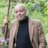# It All Adds Up to the Mark of the BeastA very learned, scholarly friend sent this to me.I summarily dismissed it as a clever meme, but utter nonsense. And then I took a closer look at the numbers using ancient secrets of numerology. My eyes were opened.

First, I assigned numbers to M A G A according to their position in the alphabet.Then, I started doing the math.

• (13 x 1) – (7 x 1) = 6
• (13 – 1 – 7) + 1 = 6
• (13/1) – (7/1) = 6
• (13 + 1) – (7 + 1) = 6
• (13 x 7) = 91.   9 – 1 – 1 – 1 = 6
• (13 – 7) x 1 x 1 = 6

6  6  6  6  6  6. Nothing but sixes! And what is the Mark of the Beast according to the Book of Revelation in the Bible? 666, duh! Hey, it’s math. I’m not just making this up. Go ahead, check my work. I could go on, but math is hard.

Except … that’s not what’s printed on the hat. Did I just do a lot of high-end math for nothing? Back to number assignment.More number crunching from left to right.

• 1 + 3 + 1 + 1 = 6
• 1 + 5 = 6
• (1 x 13) – 5 + 1 + 8 = 17. Then, 7 – 1 = 6

So, top line on the hat clearly yields 6 6 6.

Let’s take a look at the second line.

• 7 + 18 + 5 + 1 + 20 = 51. Then, 5 + 1 =
• 7 – 1 = 6
• 1 + 9 + 14 = 24. Then 2 + 4 = 6.

Not surprisingly, the bottom line of the hat also numerically yields 6 6 6.

The numbers don’t lie. Donald Trump is the Beast foretold by the Bible.Ew. The same learned scholar that sent me the first meme also sent me this Trump Pig Beast pic. Maybe he’s not so learned or scholarly after all.

## 6 thoughts on “It All Adds Up to the Mark of the Beast”

1.moonscatacombs says:

This is too weird to handle. You really sit around with that mAth? Why aren’t more talking about this mark of the beast?

Like

1.jimflanigan says:

Numbers are fun

Like

2.Bob Flowerdew (@FlowerdewBob) says:

re MAGA numerology; suggest you might recalculate using old Greek alphabet as it would have been written when this was prophecied; uppercase Mu / M was 12th (not 13th as now), upper case Alpha A was 1st, lowercase Zeta the original symbol of g was 6th letter at time (not 7th as now) plus Alpha again 12+1+6+1

Liked by 1 person

1.jimflanigan says:

Thanks for reading and your suggestion, but it’s all Greek to me.

Like